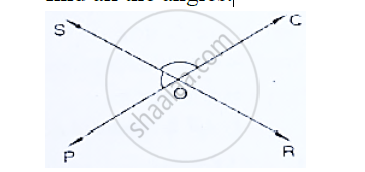# In the Below Fig, Lines Pq and Rs Intersect Each Other at Point O. If ∠Por: ∠Roq − 5 : 7, Find All the Angles. - Mathematics

In the below fig, lines PQ and RS intersect each other at point O. If ∠POR: ∠ROQ − 5 : 7,
find all the angles.#### Solution

Given ∠POR and ∠ROP is linear pair

∠POR + ∠ROP = 180°

Given that

∠POR : ∠ROP = 5 : 7

∴ ∠POR =5/12×  ´180 = 75°

Similarly ∠ROQ =   7/(5+7) ×180° = 105°

Now, ∠POS = ∠ ROQ = 105°              [ ∵ Vertically opposite angles]

∴ ∠SOQ = ∠POR = 75°                        [ ∵  Vertically opposite angles]

Concept: Concept of Parallel Lines
Is there an error in this question or solution?

#### APPEARS IN

RD Sharma Mathematics for Class 9
Chapter 10 Lines and Angles
Exercise 10.2 | Q 16 | Page 16